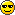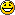Page 1 of 2

### mathematical calculation

Posted: Tue Mar 05, 2019 4:21 am
Is there a function that performs more complex mathematical calculations in LiveCode?
I tried to calculate a probability following log normal distribution or Gamma distribution, but I couldn't do it with simple function.
I also wish there are some APIs or functions to calculate integral functions.

### Re: mathematical calculation

Posted: Tue Mar 05, 2019 12:16 pm
Hello yochankim,

welcome to the forum!
I'll move this to the "Talking Livecode" forum, since this is defintively no "Announcement".

Best

Klaus

### Re: mathematical calculation

Posted: Tue Mar 05, 2019 2:41 pm
a function that performs more complex mathematical calculations
I must be a bit slow, but I don't really understand this because, as far as I know one sets up a function
to perform a specific mathematical calculation; there is no 'function' that performs mathematical
calculations as such.

For instance (and my example is not complex), if I want a list of the first 10 cube numbers I might
make this sort of thing:
function KAABA
for X=1 to 10
put (X^3) into line X of field "fOUTPUT"
next X
end KAABA
and then call the function from somewhere

although, frankly I'd not bother putting that inside a function with a name;
I'd just put it inside a "on mouseUp" statement in a button.

### Re: mathematical calculation

Posted: Tue Mar 05, 2019 3:14 pm
@Richmond.

Code: Select all

``````on mouseUp
repeat with y = 1 to 10
put y ^ 3 into line y of fld "output"
end repeat
end mouseUp ``````
@ yochankim

Anyway, there are several built-in functions that perform more complex mathematical tasks than, say, "add".
But there are no function that, as you mentioned, will integrate even a function such as "x^2 dx". This sort of thing has to built with explicit code.

What specifically did you have in mind?

Craig Newman

### Re: mathematical calculation

Posted: Tue Mar 05, 2019 3:25 pm
There is no integration method implemented in LiveCode, so normal, lognormal or gamma distributions are not "directly" doable in LC Script.
Implementing integration by some method for that would be much too slow with LC Script.
But there is a fine javaScript library that is very fast and accessible via a browser widget.

[I'll publish a statistical-methods-stack to that until the end of next week (is already 70% done).]

### Re: mathematical calculation

Posted: Tue Mar 05, 2019 5:36 pm
No: it's not: it is a bad case of my mixing up BBC BASIC with LiveCode.- -
Just thinking about the possibility makes me excited.

### Re: mathematical calculation

Posted: Tue Mar 05, 2019 6:32 pm
I tried to calculate a probability following log normal distribution or Gamma distribution, but I couldn't do it with simple function.
No, I don't suppose you can.

If you are trying to do this sort of calculation I don't think should hold you back in any way,
so my feeling is that you have to sit down with a pencil and paper and work out a number of
algorhithms that can do this sort of calculation AND then implement them in LiveCode.

If you are looking for ready-made stuff in LiveCode that will do your work for you, you
will be disappointed: but that is because LiveCode is a programming suite and not a
pre-packaged Mathematics application such as Wolfram Mathematica:

https://www.wolfram.com/mathematica/?source=nav

If you are looking for people who will do your Mathematics for you on the LiveCode Forums
you will also be disappointed.

If you are looking for help in the general direction of developing algorhithms to solve
Maths problems you should find it on the Forums and here:

http://livecode1001.blogspot.com/search ... Statistics

http://livecode1001.blogspot.com/search ... athematics

### Re: mathematical calculation

Posted: Tue Mar 05, 2019 9:53 pm
Just for fun, and to see if I remember just a little calculus and also to not work at my job for a little while, this function will integrate an algebraic expression in a field 1 and put the result into a fld 2:

Code: Select all

``````on mouseup
put integrate(fld 1,"x") into fld 2
end mouseup

function integrate arg,var
set the itemDel to var
put item 1 of arg into coeff
if coeff = 0 or coeff = 1 then put "" into coeff
put item 2 of arg into everythingElse
if everythingElse contains "^" then
set the itemDel to "^"
put word 1 of item 2 of everythingElse into expon
put word 2 of everythingElse into operator
put word 3 of everythingElse into const
else
put 1 into expon
put word 1 of everythingElse into operator
put word 2 of everythingElse into const
end if

multiply coeff by expon
if coeff = 0 then put "" into coeff
return coeff & var & "^" & expon && "/" && expon && operator && const & var && "C"
end integrate``````
It is purely brute-force, and, er, somewhat limited in scope.

But this is what you would have to do to create a library of math functions.

Craig

### Re: mathematical calculation

Posted: Wed Mar 06, 2019 5:06 am
Thank you,richmond62!
You showed me the reality of LiveCode and its potential also.
Anyway can I get the source code of the statistical program in the "livecode1001...Statistics" site?
richmond62 wrote:
Tue Mar 05, 2019 6:32 pm

If you are looking for help in the general direction of developing algorhithms to solve
Maths problems you should find it on the Forums and here:

### Re: mathematical calculation

Posted: Wed Mar 06, 2019 5:17 am
Craig,

Thank you for sharing your idea of integration. That was what I looking for.
If you don't mind, can I know how can I describe a mathematical expression in field 1?
Just showing an example is enough to understand your function.
Thank you so much.
dunbarx wrote:
Tue Mar 05, 2019 9:53 pm
Just for fun, and to see if I remember just a little calculus and also to not work at my job for a little while, this function will integrate an algebraic expression in a field 1 and put the result into a fld 2:

Code: Select all

``````on mouseup
put integrate(fld 1,"x") into fld 2
end mouseup

function integrate arg,var
set the itemDel to var
put item 1 of arg into coeff
if coeff = 0 or coeff = 1 then put "" into coeff
put item 2 of arg into everythingElse
if everythingElse contains "^" then
set the itemDel to "^"
put word 1 of item 2 of everythingElse into expon
put word 2 of everythingElse into operator
put word 3 of everythingElse into const
else
put 1 into expon
put word 1 of everythingElse into operator
put word 2 of everythingElse into const
end if

multiply coeff by expon
if coeff = 0 then put "" into coeff
return coeff & var & "^" & expon && "/" && expon && operator && const & var && "C"
end integrate``````
It is purely brute-force, and, er, somewhat limited in scope.

But this is what you would have to do to create a library of math functions.

Craig

### Re: mathematical calculation

Posted: Wed Mar 06, 2019 5:19 am
I will look forward your product. Thank you for the valuable comment!
[-hh] wrote:
Tue Mar 05, 2019 3:25 pm
There is no integration method implemented in LiveCode, so normal, lognormal or gamma distributions are not "directly" doable in LC Script.
Implementing integration by some method for that would be much too slow with LC Script.
But there is a fine javaScript library that is very fast and accessible via a browser widget.

[I'll publish a statistical-methods-stack to that until the end of next week (is already 70% done).]

### Re: mathematical calculation

Posted: Wed Mar 06, 2019 5:30 am
@Craig

Thank you for your answer. I am just looking the integration function, but, I have to develop it by myself as you write.
If somebody develop a library supporting the mathematical function, I will buy it!
But, unfortunately, I will develop the code for my personal purpose.
Anyway, thank you Craig!dunbarx wrote:
Tue Mar 05, 2019 3:14 pm
@Richmond.

Code: Select all

``````on mouseUp
repeat with y = 1 to 10
put y ^ 3 into line y of fld "output"
end repeat
end mouseUp ``````
@ yochankim

Anyway, there are several built-in functions that perform more complex mathematical tasks than, say, "add".
But there are no function that, as you mentioned, will integrate even a function such as "x^2 dx". This sort of thing has to built with explicit code.

What specifically did you have in mind?

Craig Newman

### Re: mathematical calculation

Posted: Wed Mar 06, 2019 5:31 am
@richmond62

I am trying to develop a function for my program.richmond62 wrote:
Tue Mar 05, 2019 2:41 pm
a function that performs more complex mathematical calculations
I must be a bit slow, but I don't really understand this because, as far as I know one sets up a function
to perform a specific mathematical calculation; there is no 'function' that performs mathematical
calculations as such.

For instance (and my example is not complex), if I want a list of the first 10 cube numbers I might
make this sort of thing:
function KAABA
for X=1 to 10
put (X^3) into line X of field "fOUTPUT"
next X
end KAABA
and then call the function from somewhere

although, frankly I'd not bother putting that inside a function with a name;
I'd just put it inside a "on mouseUp" statement in a button.

### Re: mathematical calculation

Posted: Wed Mar 06, 2019 5:33 am
Dear Klaus,

I am not familiar with any kind of forum websites. I am sorry for that.
Next time, I will post my opinions or questions on the right place.
Thank you!!
Klaus wrote:
Tue Mar 05, 2019 12:16 pm
Hello yochankim,

welcome to the forum!
I'll move this to the "Talking Livecode" forum, since this is defintively no "Announcement".

Best

Klaus

### Re: mathematical calculation

Posted: Wed Mar 06, 2019 6:11 am
I have stack that I uploaded to LiveCode Sample Stack called Coup de Graph. It may give you some ideas.

Cheers,
Roger